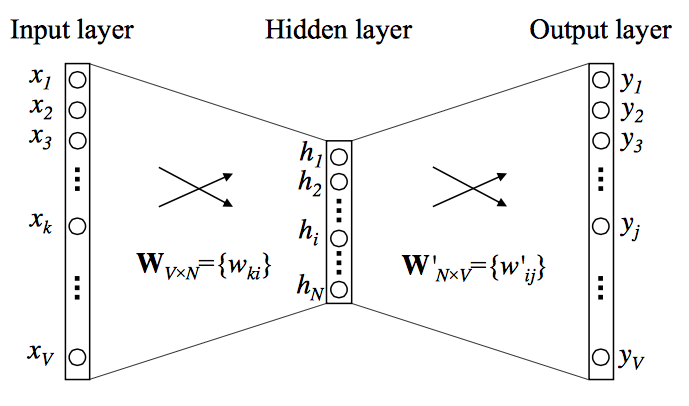One of my personal goals is to better understand deep learning. Word2vec, an algorithm developed by Google, is one of the simplest (but still not simple!) forms of deep learning. Deep Learning is a type of machine learning when there is at least one hidden layer. Word2vec is an algorithm that develops vectors for words based purely on the assumption that similar words are often used in similar ways. By running words through this algorithm, you can find a vector for a word, and then take the cosine between 2 vectors to find how similar the words are…so cool! There are 2 ways that word2vec can be implemented.
• CBOW (Continuous Bag of Words) - The algorithm is given a context (n words before and after the word), and optimizes for the middle word. This is the strategy that I used and implemented.
• Skip Gram - The algorithm is given the middle word and optimizes for the context

The gist of the CBOW word2vec algorithm is the following:
1. Start with an enormous corpus. An often used corpus is called text8 (http://mattmahoney.net/dc/textdata and search for text8.zip). I copied it into corpus.txt by using `head -1 text8 > corpus.txt`. This command puts everything on the first line of the text8 file into corpus.txt. text8 is a huge 1 line file, so it works out.
2. Assign random values between [-1, 1] for all of the entries in W, W’, and h.
3. Generate a map D and DPrime. D is a map with every word in the vocabulary as the key and with a list of each context that it appears in as the value. DPrime is a map where each key is a word and its value is a list of some contexts that DO NOT appear in the text for that word. When Google was developing word2vec, they found that it was important to both optimize words and contexts that SHOULD go together, as well as “teach" the network about words and contexts that should NEVER go together.
4. Run each context in D and DPrime through the network below TRAINING_ITERATIONS times.

The architecture of the one hidden layer network:• There are V unique words in the vocabulary and the hidden layer (single dimension vector h) has length N
• With CBOW, vector x becomes a vector with a 1 in all places where the context word exists and a 0 everywhere else.
• In my script, I arbitrarily used an N of size 100. All other constants that I chose are at the top of my script.js file as well.
1. In each iteration of the learning loop:
1. Create intermediate vector Vc:
1. The x vector is 1 at the indices of the context and 0 everywhere else. Therefore, when you multiply W transpose * X, the output will be the columns of W transpose that have the indices of the context. This is equivalent to the rows of W that have the indices of the context words. Vc is a vector with N rows and 1 column because that is the output of doing W transpose * X
2. Create intermediate vector Vw:
1. Vw is the column of the W’ matrix (or the row of the W’ transpose matrix) that corresponds to the middle word
3. Find the intermediate output. This is the output that you are optimizing in each iteration of the loop. The following equations come from taking the derivative of the https://en.wikipedia.org/wiki/Maximum_likelihood">Maximum Likelihood Estimation.
1. If we are looking at a middle word and context that is not in the corpus:
1. Log(1 / (1 + e^(Vw x Vc)))
2. If we are looking at a middle word and context that is in the corpus:
1. Log(1 / (1 + e^(Vw x Vc x (-1))))
4. Use gradient ascent to update each element in the W and W’ matrix that was affected by this iteration. The rows of W that are affected are the rows in the context, and the column of W’ that is affected is the column corresponding to the middle word. Gradient ascent is used to maximize a function, and in this case we are trying to maximize the likelihood that the middle word is the word in between the context. Gradient descent is used when trying to minimize a function.

In every epoch of the loop, I update all of the word vectors by writing to a csv file, as well as writing a `hello.txt` that finds the 10 words that are most similar to the word hello. We find word similarity by finding the 10 words with the largest cosine similarity.

I hope my JavaScript is simple and easy to understand 😃 https://github.com/harrisosserman/word2vec

The best materials that I found on the topic are these 2 YouTube videos from University of Waterloo Professor Ali Ghodsi. Thanks so much for your lectures!

After writing the algorithm, I enjoyed reading this paper to tune and optimize my word vector output: https://levyomer.files.wordpress.com/2015/03/improving-distributional-similarity-tacl-2015.pdf